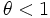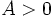# Elliott-Halberstam conjecture

## Statement

Fora natural number andan integer relatively prime to, consider the modular prime-counting function, which counts the number of prime numbers less than or equal tothat are congruent tomodulo. Letbe the prime-counting function atandthe Euler phi-function of. Consider the expression:The intuition here is that the primes should be roulghy equally distributed between the various congruence classes modulo, so the expected number of primes in each congruence class is. We are interested in the largest of the deviations from this expected value.

Then, the Elliott-Halberstam conjecture states that for everyandthere exists a constantsuch that## Relation with other facts/conjectures

Name Statement Status
Bombieri-Vinogradov theorem Elliott-Halberstam conjecture holds forProved assuming generalized Riemann hypothesis